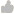Support Center > Knowledge base> Article: What does the Index Score for each Training Program mean? How was the score calculated?

# What does the Index Score for each Training Program mean? How was the score calculated?

Article ID: 98580Question
What does the Index Score for each Training Program mean? How was the score calculated?